# M5-S5: Practical Investigations of Le Chatelier's Principle

• ### Conduct practical investigations to analyse the reversibility of chemical reactions, for example:

– cobalt (II) chloride hydrated and dehydrated
– iron (III) nitrate and potassium thiocyanate
– burning magnesium
– burning steel wool (ACSCH090)

• ### Investigate the effects of temperature, concentration, volume and/or pressure on a system at equilibrium and explain how Le Chatelier’s principle can be used to predict such effects, for example:

- heating cobalt (II) chloride hydrate
- interaction between nitrogen dioxide and dinitrogen tetroxide
- iron(III) thiocyanate and varying concentration of ions (ACSCH095)

### Cobalt (II) Chloride in Hydrated & Dehydrated Forms

• Cobalt (II) chloride, when dissolved in water, forms an equilibrium between a hydrated form and dehydrated form. The hydrated form involves the cobalt ion being compounded to 6 water molecules while the dehydrated form does not.

$${{Co(H_2O)_6}^{2+}}_{(aq)} + \; 4{Cl^-}_{(aq)}\; \rightleftharpoons \;{{CoCl_{4}}^{2-}}_{(aq)} + \; 6H_2O_{(l)} \;\;\; \Delta{H}>0$$Figure: Two solutions of cobalt chloride containing different concentrations of hydrated and dehydrated forms. Left (pink) contains higher concentration of hydrated form while the solution on the right (blue) contains higher concentration of the dehydrated form.

• Hydrated cobalt ion displays a pink colour whilst cobalt (II) chloride (dehydrated) displays a blue colour. This chemical equilibrium can be easily monitored by the colour change between pink and blue.
• Pressure and volume do not affect this reaction as there are no gaseous reactants or products.

Concentration

• Adding HCl to the solution will initially dilute the solution and make the colour paler. The addition also increases [Cl-] which in turn increases the rate of forward reaction and shifts the chemical equilibrium to the right. Solution turns blue.

$${Ag^+}_{(aq)} + {Cl^-}_{(aq)} \rightarrow AgCl_{(s)}$$

• Adding silver nitrate (AgNO3): Ag+ forms white precipitate with Cl- which decreases [Cl-]. The solution quickly turns cloudy white. This change increases the rate of reverse reaction and shifts the chemical equilibrium to the left. Solution turns pink. The pink colour will only be visible after the white precipitate settles to the bottom.

• Adding distilled water (de-ionised water) increases the volume of the solution, and more importantly increases the amount of H2O in the system. This initially dilutes the solution, making the colour paler. By Le Chatlier’s principle, an increase in [H2O] increases the rate of reverse reaction and shifts the chemical equilibrium to the left. Solution turns light pink.

Temperature

• Heating the solution in a water bath drives the forward reaction (endothermic). This increases the concentration of hydrated form of cobalt ion. Solution turns blue.
• Cooling the solution in ice drives the reverse reaction (exothermic). This increases the concentration of dehydrated form of cobalt ion. Solution turns pink.

### Iron (III) Nitrate & Potassium Thiocyanate

• When iron (III) nitrate and potassium thiocyanate are mixed in solution, an equilibrium mixture of Fe3+, NCS- and the complex ion FeNCS2+ is formed:

$${Fe{(NO_3)}_3}_{(aq)} + \; KNCS_{(aq)} \rightleftharpoons \; {FeNCS^{2+}}_{(aq)} + \; KNO_{3(aq)} \;\;\; \Delta{H}<0$$

• Net ionic equation:

$${Fe^{3+}}_{(aq)} + \; {NCS^-}_{(aq)} \rightleftharpoons \; {FeNCS^{2+}}_{(aq)} \;\;\; \Delta{H}<0$$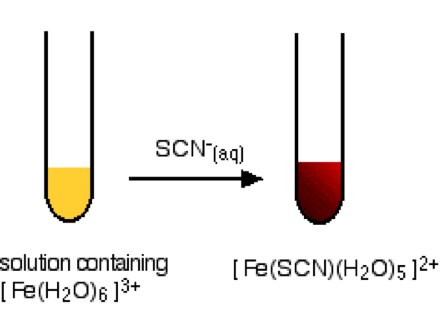Figure: a similar reaction that demonstrates the colour change of Fe3+’s conversion to form Fe(SCN)2+

• Fe3+ is yellow and FeNCS2+ is blood red.
• Potassium ion and nitrate ion are spectator ions and are not part of the chemical equilibrium.
• Pressure and volume do not affect this reaction as there are no gaseous reactants nor products.

Concentration• Tube A is a reference tube – used for comparison purposes.
• Tube B: Addition of Na2HPO4: hydrogen phosphate ions react with Fe3+ to form complex ions:

$${Fe^{3+}}_{(aq)} + \; {{HPO_4}^{2-}}_{(aq)} \rightarrow \; {{FeHPO_4}^+}_{(aq)}$$

This reduces [Fe3+] which in turn increases the rate of backward reaction and shifts the chemical equilibrium to the left. Solution turns less blood red, paler yellow or colourless.

• Tube C: Addition of KNCS crystals increases concentration of reactants of forward reaction. This increases the rate of forward reaction and shifts the equilibrium to the right. Solution turns more blood red
• Tube D: Addition of Fe(NO3)3 increases the concentration of reactants of the forward reaction. This will initially make the solution more yellow as [Fe3+] increases. However, by Le Chatelier’s principle, this change in turn increases the rate of forward reaction and shifts the equilibrium to the right. Solution turns more blood red.
• Addition of SnCl2 (or any form of Sn2+) reduces Fe3+ to Fe2+. Reduction of Fe3+ is possible because Sn2+ is a stronger reductant.

$$Sn^{2+} + 2Fe^{3+} \rightarrow Sn^{4+} + 2Fe^{2+}$$

Reduction decreases [Fe3+] which in turn increases the rate of reverse reaction and shifts the chemical equilibrium to the left. Solution turns less red and more yellow.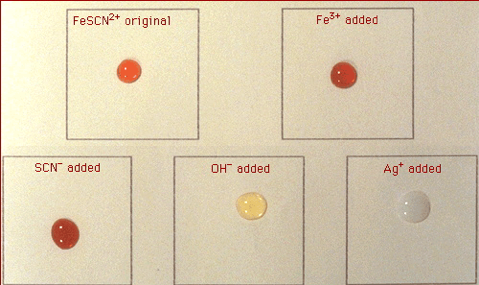Figure: colour analysis of Fe3+, NCS- and the complex ion FeNCS2+ equilibrium.

• Adding silver nitrate (AgNO3): Ag+ forms white precipitate with NCS- which decreases [NCS-]:
$${Ag^+}_{(aq)} + {NCS^-}_{(aq)} \rightarrow AgNCS_{(s)}$$

This increases the rate of backward reaction and shifts the chemical equilibrium to the left. Solution turns cloudy white. Precipitate will settle to the bottom of the tube if left undisturbed for a long time.

Temperature

• The formation of iron (III) thiocyanate complex ion is an exothermic reaction. This means the forward reaction is favoured at a low temperature.Figure: effect of temperature on the dynamic equilibrium of iron thiocyanate solution.

• When the solution is placed in ice-cold water, more iron thiocyanate ion is formed. This causes the solution to appear more blood red.

As the temperature of water increases, the reverse reaction becomes more favoured. This causes the solution to appear less blood red and start to adopt a yellow colour.

### Nitrogen Dioxide & Dinitrogen Tetroxide

• The equilibrium mixture of NO2 and N2O4 is investigated using a gas syringe (closed system).

$$2NO_{2(g)} \rightleftharpoons N_2O_{4(g)} \;\;\; \Delta H=-58 \; kJ mol^{-1}$$

• Nitrogen dioxide (NO2) is brown in colour while dinitrogen tetroxide (N2O4) is colourless

Pressure & Volume• The effect of pressure and volume can be tested by pushing or pulling the plunger of the syringe to increase and decrease pressure respectively. Increasing the pressure directly increases the collision rate between gas molecules. This in turn increases the rate of reaction.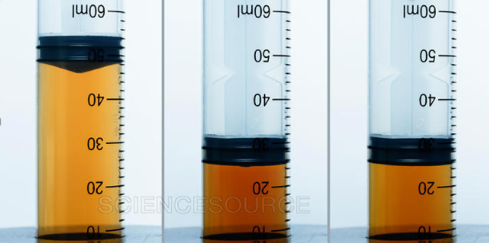Figure: volume of syringe decreases by half which in turn doubles concentration. Solution slowly becomes less brown as the chemical equilibrium shifts to the right (Le Chatelier’s principle).

• Pushing the plunger increases pressure and decreases volume. This increases the concentration of both gases. Solution initially turns brown quickly. However, since there are more moles of gases on the reactant side (NO2), the forward reaction rate is increased more than the reverse as a result of this change. By Le Chatelier’s principle, some of NO2 is consumed to form N2O4. This is represented by the gradual decrease in the solution’s brown appearance.

Increasing the pressure on the system favours the reaction that would produce less moles of gases. Chemical equilibrium shifts to the side with less moles of gases (N2O4) and solution turns less brown.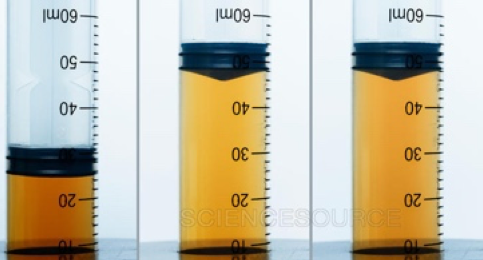Figure: volume of syringe doubles which in turn halves concentration. Solution slowly becomes browner as the chemical equilibrium shifts to the left (Le Chatelier’s principle).

• Pulling the plunger decreases pressure and increases volume. This decreases the concentration of both gases. Solution initially turns less brown quickly. The forward reaction rate is decreased more than the reverse as a result of this change. By Le Chatelier’s principle, some of N2O4 is consumed to form NO2. This is represented by the gradual increase in the solution’s brown appearance.

Reducing the pressure on the system favours the reaction that would produce more moles of gases. Chemical equilibrium shifts to the side with more moles of gases (NO2) and solution turns browner.

TemperatureHeating the solution in warm water turns it brown as the formation of NO2 is endothermic.

• Heating the solution increases rate of reverse reaction (endothermic). This increases [NO2] and turns the solution browner.Icing the solution turns it pale as the formation of N2O4 is exothermic.

• Cooling the solution in ice reduces the rate of the reverse reaction (endothermic) more than the forward reaction. This means it favours the forward reaction (exothermic). This increases [N2O4] and causes the solution to turn pale.

### Combustion of Magnesium

• Burning magnesium is an example of metal combustion reaction which is highly exothermic. It releases energy in the form of light and heat.

$$2Mg_{(s)} + O_{2(g)} \rightarrow 2MgO_{(s)}\;\;\; \Delta{H}<0$$Figure: burning a magnesium strip generates light energy. It is an irreversible process.

• Flame (heat energy) is required to overcome the relatively small activation energy barrier of the reaction.
• Burning magnesium goes to completion (until magnesium strips are fully converted into magnesium oxide). It is an irreversible reaction because magnesium oxide is very stable at standard conditions. The activation energy of the reverse reaction (endothermic) is also much larger.

### Burning Steel Wool

• Similar to magnesium, burning steel wool (made of mostly iron) is an example of exothermic, irreversible reaction.

$$4Fe_{(s)} + 3O_{2(g)} \rightarrow 2Fe_2O_{3(s)}\;\;\; \Delta{H}<0$$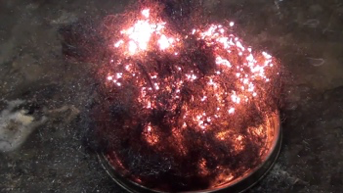Burning steel wool is similar to burning magnesium. The formation of iron oxide is an irreversible process.

• The activation energy of the reverse reaction is relatively large under standard conditions.

Previous section: Le Chatelier's Principle

﻿Next section: ﻿Further Practical Investigations of Le Chatelier's Principle